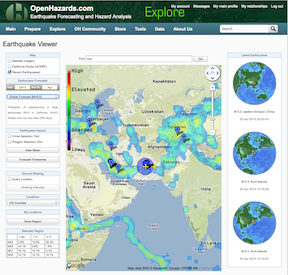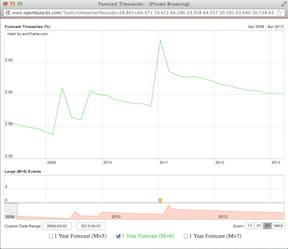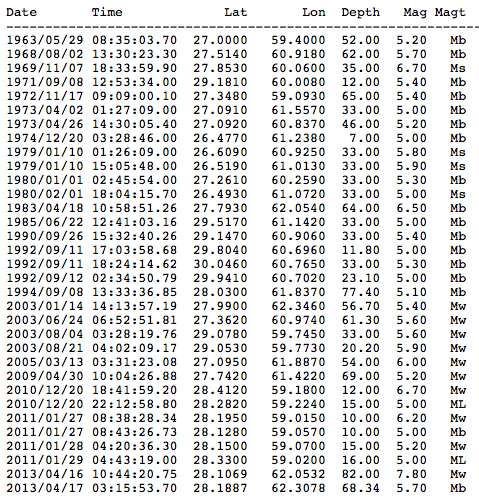# And a Third Earthquake: The M7.8 Iran Earthquake of 4/16/2013On monday April 16, a M7.8 earthquake struck near the borders of Iran, Pakistan and India.  Continuing on the theme of the last blog, the lack of small earthquake data in some regions of the globe is again illustrated.   The first figure below is a screen shot of the affected region.  Again, the radius of the circle is 243 km = 150 miles.The second figure below is the forecast probability timeseries since 2008 for an M>6 earthquake. The computed probabilities are miniscule, a tiny fraction of 1%.  This can't be correct, either because the method is wrong, or doesn't apply to this region, or because there is little to no data for small earthquakes in the ANSS catalog.   As explained in the previous blog, the method works by counting the small earthquakes since the last large earthquake.  The famous Gutenberg-Richter relation says that, if the b parameter =1, 1000 M>3 earthquakes must occur on average for ever 1 M>6 earthquake.

While we can't conclude which of these reasons is the best answer, we can at least check the catalog completeness.  So the last figure 3 is the result of an ANSS catalog search in the blue circle region of the screenshot figure 1.  We see that since 1963, the catalog contains only 33 earthquakes having a magnitude greater than M5.  Since 2005, there have been only 9 such events (apparently).  Of these, 6 were 5<M<6, 2 more were 6<M<7, and then there was the 1 event last monday of M7.8.  This distribution of earthquake magnitudes does not reflect a GR distribution of earthquakes.

Figure 2:  Forecast probability time series.Figure 3.  Results from the ANSS catalog search for the blue circular region of figure 1.Risk Alert